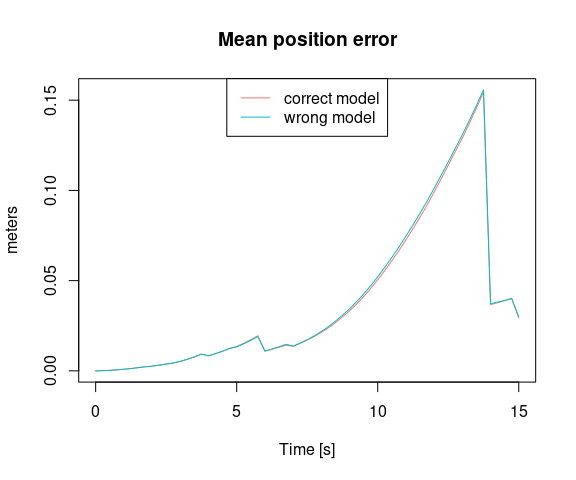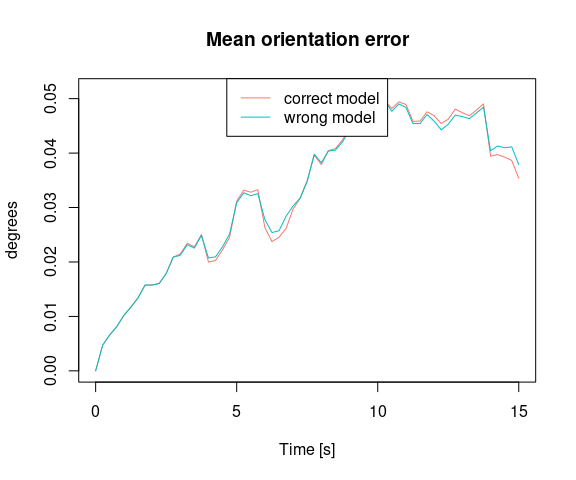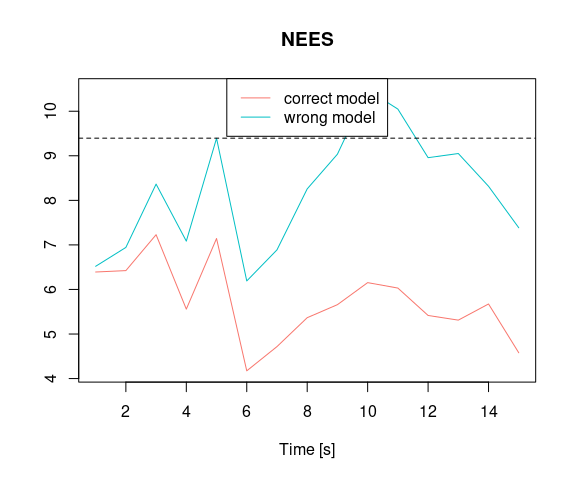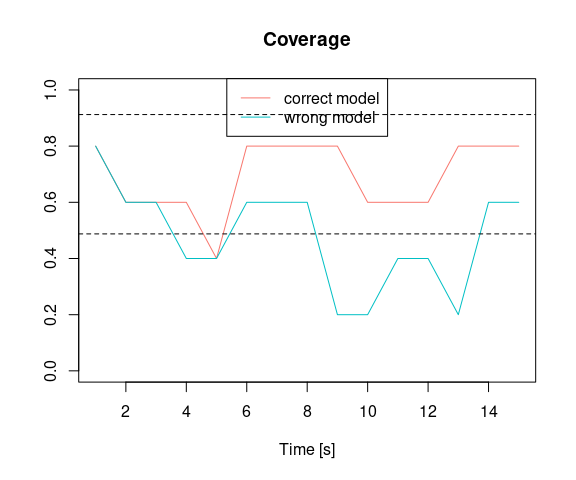# Compare Models

We load the R package navigation

library(navigation)

We load the data lemniscate_traj_ned

data("lemniscate_traj_ned") # trajectory in proper format as shown bellow
head(lemniscate_traj_ned)
##         t          x          y z         roll     pitch_sm       yaw
## [1,] 0.00 0.00000000 0.00000000 0 0.0000000000 0.000000e+00 0.7853979
## [2,] 0.01 0.05235987 0.05235984 0 0.0001821107 8.255405e-05 0.7853971
## [3,] 0.02 0.10471968 0.10471945 0 0.0003642249 1.650525e-04 0.7853946
## [4,] 0.03 0.15707937 0.15707860 0 0.0005463461 2.474976e-04 0.7853905
## [5,] 0.04 0.20943890 0.20943706 0 0.0007284778 3.298918e-04 0.7853847
## [6,] 0.05 0.26179819 0.26179460 0 0.0009106235 4.122374e-04 0.7853773

We make the trajectory object

traj <- make_trajectory(data = lemniscate_traj_ned, system = "ned")
class(traj)
##  "trajectory"

We then define a timing object

timing <- make_timing(
nav.start = 0, # time at which to begin filtering
nav.end = 15,
freq.imu = 100, # frequency of the IMU, can be slower wrt trajectory frequency
freq.gps = 1, # gnss frequency
freq.baro = 1, # barometer frequency (to disable, put it very low, e.g. 1e-5)
gps.out.start = 8, # to simulate a GNSS outage, set a time before nav.end
gps.out.end = 13
)

We define the sensor model for generating sensor errors.

snsr.mdl <- list()
acc.mdl <- WN(sigma2 = 5.989778e-05) + AR1(phi = 9.982454e-01, sigma2 = 1.848297e-10) + AR1(phi = 9.999121e-01, sigma2 = 2.435414e-11) + AR1(phi = 9.999998e-01, sigma2 = 1.026718e-12)
gyr.mdl <- WN(sigma2 = 1.503793e-06) + AR1(phi = 9.968999e-01, sigma2 = 2.428980e-11) + AR1(phi = 9.999001e-01, sigma2 = 1.238142e-12)

snsr.mdl$imu <- make_sensor(name = "imu", frequency = timing$freq.imu, error_model1 = acc.mdl, error_model2 = gyr.mdl)

We define the stochastic model for the GPS errors considering a RTK-like GNSS system

gps.mdl.pos.hor <- WN(sigma2 = 0.025^2)
gps.mdl.pos.ver <- WN(sigma2 = 0.05^2)
gps.mdl.vel.hor <- WN(sigma2 = 0.01^2)
gps.mdl.vel.ver <- WN(sigma2 = 0.02^2)
snsr.mdl$gps <- make_sensor( name = "gps", frequency = timing$freq.gps,
error_model1 = gps.mdl.pos.hor,
error_model2 = gps.mdl.pos.ver,
error_model3 = gps.mdl.vel.hor,
error_model4 = gps.mdl.vel.ver
)

We define the stochastic model for the barometer

baro.mdl <- WN(sigma2 = 0.5^2)
snsr.mdl$baro <- make_sensor(name = "baro", frequency = timing$freq.baro, error_model1 = baro.mdl)

We then define the stochastic model for the sensor error, here we configure the EKF to have the same model as for data generation (ideal setup).

KF.mdl <- list()

KF.mdl$imu <- make_sensor(name = "imu", frequency = timing$freq.imu, error_model1 = acc.mdl, error_model2 = gyr.mdl)
KF.mdl$gps <- snsr.mdl$gps
KF.mdl$baro <- snsr.mdl$baro

We then define a wrong model with respect to the data generation process (only composed of the white noise part)

wrong_acc.mdl <- WN(sigma2 = 5.989778e-05)
wrong_gyr.mdl <- WN(sigma2 = 1.503793e-06)
wrong_KF.mdl <- list()
wrong_KF.mdl$imu <- make_sensor(name = "imu", frequency = timing$freq.imu, error_model1 = wrong_acc.mdl, error_model2 = wrong_gyr.mdl)
wrong_KF.mdl$gps <- snsr.mdl$gps
wrong_KF.mdl$baro <- snsr.mdl$baro

We perform the navigation Monte Carlo simulation considering the correct stochastic model.

num.runs <- 5 # number of Monte-Carlo simulations
traj.ref = traj,
timing = timing,
snsr.mdl = snsr.mdl,
KF.mdl = KF.mdl,
num.runs = num.runs,
noProgressBar = TRUE,
PhiQ_method = "1",
parallel.ncores = 1,
P_subsampling = timing$freq.imu, compute_PhiQ_each_n = 50 ) # keep one covariance every second We perform the navigation Monte Carlo simulation considering the wrong stochastic model. wrong_res <- navigation( traj.ref = traj, timing = timing, snsr.mdl = snsr.mdl, KF.mdl = wrong_KF.mdl, # < here the model is the wrong one num.runs = num.runs, noProgressBar = TRUE, PhiQ_method = "1", parallel.ncores = 1, P_subsampling = timing$freq.imu,
compute_PhiQ_each_n = 50
) # keep one covariance every second

We compute statistics on navigation performance for the navigation simulation that considered the correct stochastic model and for the navigation simulation that considered the wrong stochastic model.

pe_res <- compute_mean_position_err(res, step = 25)
pe_wrong_res <- compute_mean_position_err(wrong_res, step = 25)

oe_res <- compute_mean_orientation_err(res, step = 25)
oe_wrong_res <- compute_mean_orientation_err(wrong_res, step = 25)
nees <- compute_nees(res, step = timing$freq.imu) wrong_nees <- compute_nees(wrong_res, step = timing$freq.imu)

coverage <- compute_coverage(res, alpha = 0.7, step = timing$freq.imu) wrong_coverage <- compute_coverage(wrong_res, alpha = 0.7, step = timing$freq.imu)

We compare results

plot(pe_res, pe_wrong_res, legend = c("correct model", "wrong model"))plot(oe_res, oe_wrong_res, legend = c("correct model", "wrong model"))plot(nees, wrong_nees, legend = c("correct model", "wrong model"))plot(coverage, wrong_coverage, legend = c("correct model", "wrong model"))